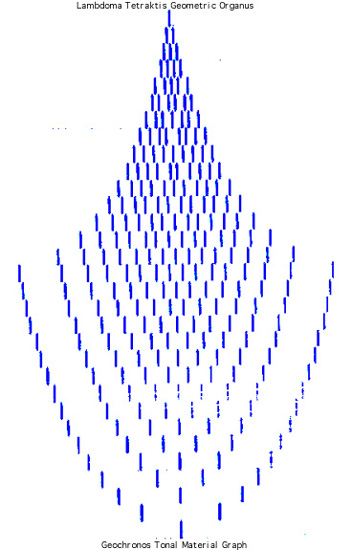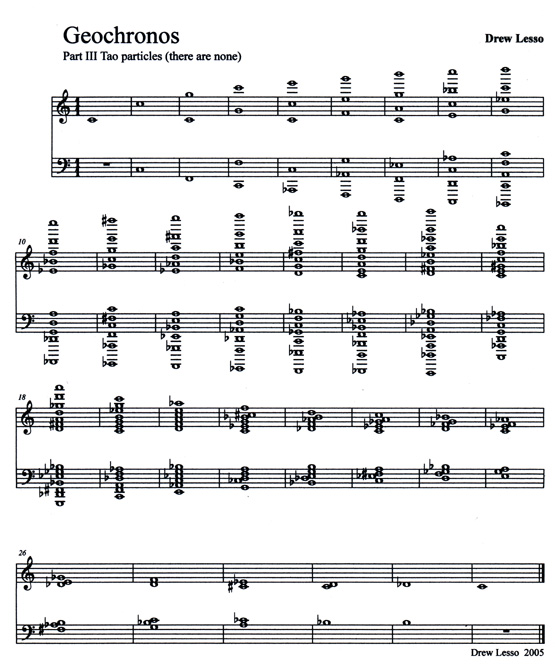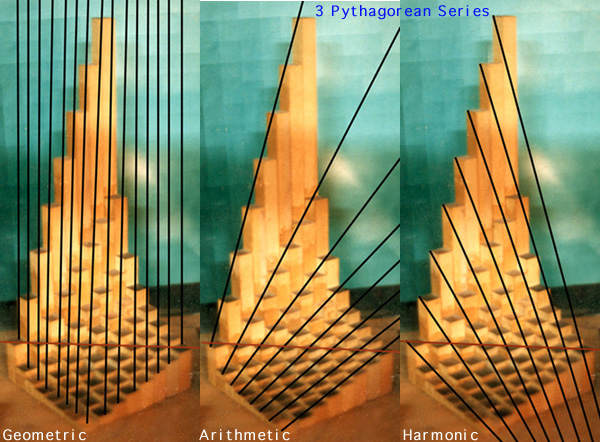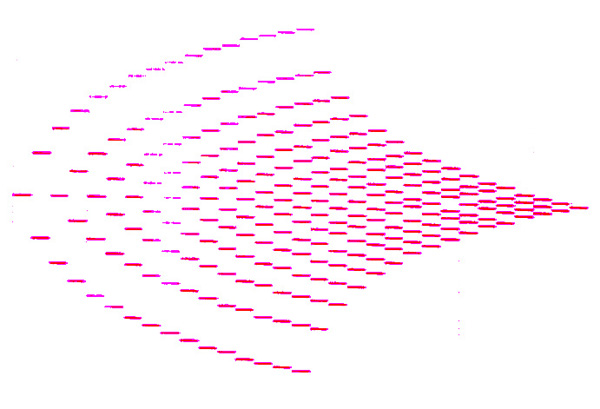# Tao Particles, GeochronosLambdoma geometric space lends itself to the construction of lush chords. In Geochronos Part III, Tao Particles my algorithm sorts all 256 particles of a 16X16 Lambdoma. These geometric diagonals are also transposed into all 12 keys. All the chords were placed by hand from a notation program by following the output of my algorithmic sort. Rhythmic values are determined directly from ratio positions in the Lambdoma. What's interesting is that there are more than twenty trillion chord sets in a 16X16 Lambdoma configuration, leaving a myriad array of chords in the Geochronos tone-space untouched. There are no Tao Particles here!1 REM Tao Particles (there are none) Part III Geochronos
2 REM Counterpoint Generator

S2TT:
3 REM Series 2 Top/Top (2 to 9-- / 16-- to 9)
4 REM (x to y-->1 / z-x to y=3)
5 LET y = 9
6 LET z = 18
7 WHILE y > 1
10 FOR x = 2 TO y
20 LPRINT x,z-x
23 IF x = y THEN LET z = z -1
25 IF x = y THEN LET y = y-1
30 NEXT x
35 LPRINT
40 LPRINT
45 LPRINT
50 WEND

REM The output of this program is used for geometric chord selection; to be placed by hand, no sequencer used here.